## Example Questions

← Previous 1 3 4 5

### Example Question #53 : Trigonometry

What is the period of 2sin(4Θ)?None of the answers are correct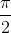Explanation:

The period of sinΘ is 2Π, so we set the new angle equal to the base period of 2Π and solve for Θ.

So 4Θ = 2Π and Θ = Π/2.

### Example Question #1 : Sine

A function with period P will repeat on intervals of length P, and these intervals are referred to as periods.

Find the period of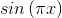.Explanation:

For the function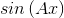the period is equal to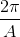in this case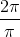which reduces to.

### Example Question #55 : Trigonometry

A function with period P will repeat on intervals of length P, and these intervals are referred to as periods.

Find the period of the function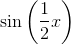.Explanation:

For the functionthe period is equal toin this case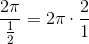which reduces to.

### Example Question #56 : Trigonometry

What is the period of the function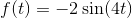?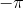Explanation:

To find the period of Sine and Cosine functions you use the formula: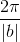wherecomes from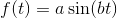. Looking at our formula you see b is 4 so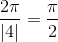### Example Question #57 : Trigonometry

What is the period of the given trigonometric function: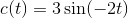. Leave your answer in terms of, simplify all fractions.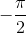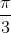Explanation:

To find the period of a sine, cosine, cosecant, or secant funciton use the formula: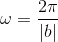wherecomes from the general formula: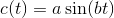. We see that for our equation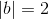and so the period iswhen you reduce the fraction.

### Example Question #58 : Trigonometry

Find the period of the following formula: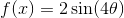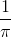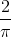Explanation:

To find period, simply remember the following formula: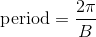where B is the coefficient in front of x. Thus,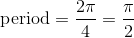### Example Question #59 : Trigonometry

Find the domain of the function: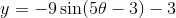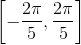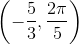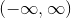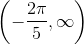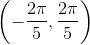Explanation:

The functionis related to the parent function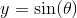, which has a domain of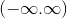.

The value of theta forhas no restriction and is valid for all real numbers.

The answer is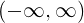.

### Example Question #60 : Trigonometry

What is the domain of the given trigonometric function: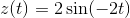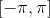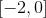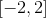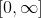Explanation:

For both Sine and Cosine, since there are no asymptotes like Tangent and Cotangent functions, the function can take in any value for. Thus the domain is:### Example Question #1 : How To Find The Range Of The Sine

Which of the following statements is (are) true:

I. The domain of the tangent function is all real numbers

II. The range of the sine function is all real numbers

III. The periods of the tangent, sine, and cosine functions are the same.

II and III only

I and II only

I only

none of the above

II only

II only

Explanation:

The domain of the tangent function does not include any values of x that are odd multiples of π/2 .

The range of the sine function is from [-1, 1].

The period of the tangent function is π, whereas the period for both sine and cosine is 2π.

### Example Question #62 : Trigonometry

Which of the following represents a sine wave with a range of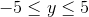?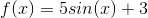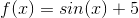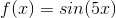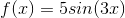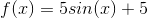The range of a sine wave is altered by the coefficient placed in front of the base equation. So, if you have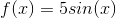, this means that the highest point on the wave will be atand the lowest at.  However, if you then begin to shift the equation vertically by adding values, as in,, then you need to account for said shift.  This would make the minimum value to beand the maximum value to be. For our question, then, it is fine to use. Thefor the function parameter only alters the period of the equation, making its waves "thinner."